# Understanding Sensor Fusion and Tracking, Part 3: Fusing a GPS and IMU to Estimate Pose

Summary
Questions Covered
Why It Matters
x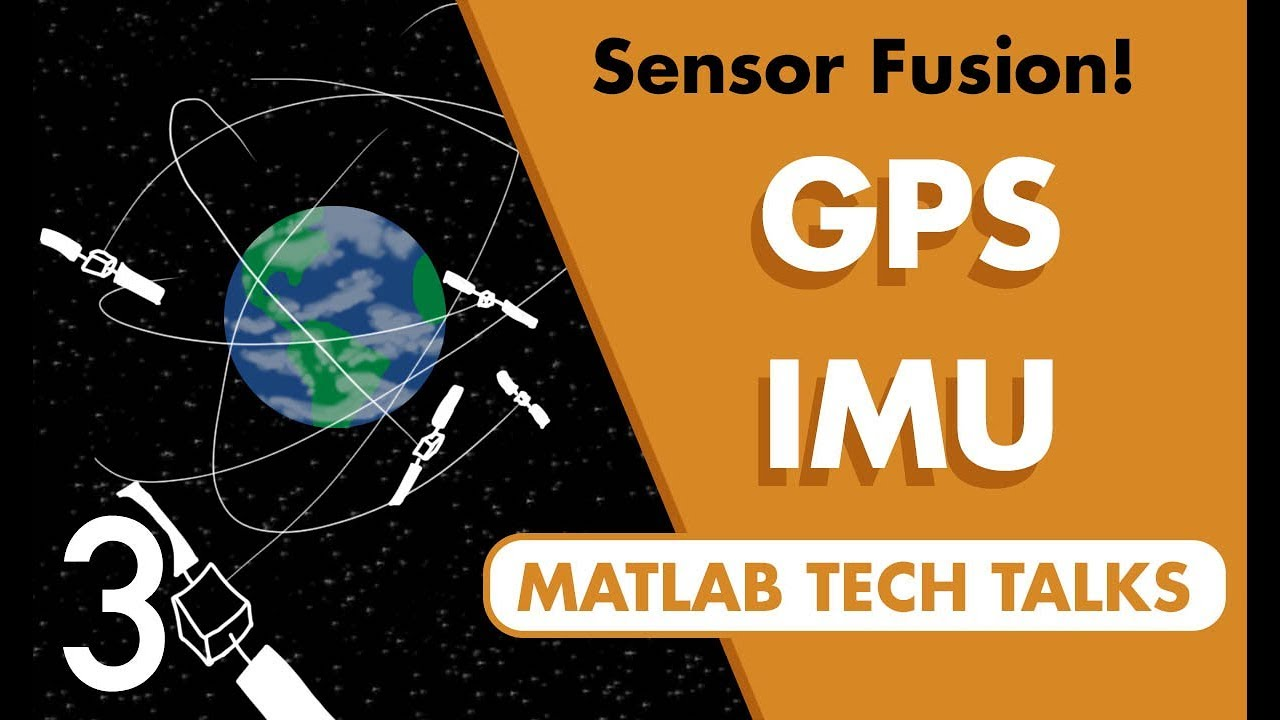Let's continue our discussion on using sensor fusion for positioning and localization. In the last video, we combined the sensors in an IMU to estimate an object's orientation and showed how the absolute measurements of the accelerometer and magnetometer were used to correct the drift from the gyro. Now in this video, we're going to do sort of a similar thing, but we're going to add a GPS sensor. Gps can measure position and velocity, and so in this way we can extend the fusion algorithm to estimate them as well. And just like the last video, the goal is not to fully describe the fusion algorithm. It's again too much for one video. Instead, I mostly want to go over the structure of the algorithm and show you visually how each sensor contributes to the final solution, so you have a more intuitive understanding of the problem. So I hope you stick around for it. I'm Brian, and welcome to a MATLAB Tech Talk now. Show more

Show lessShow less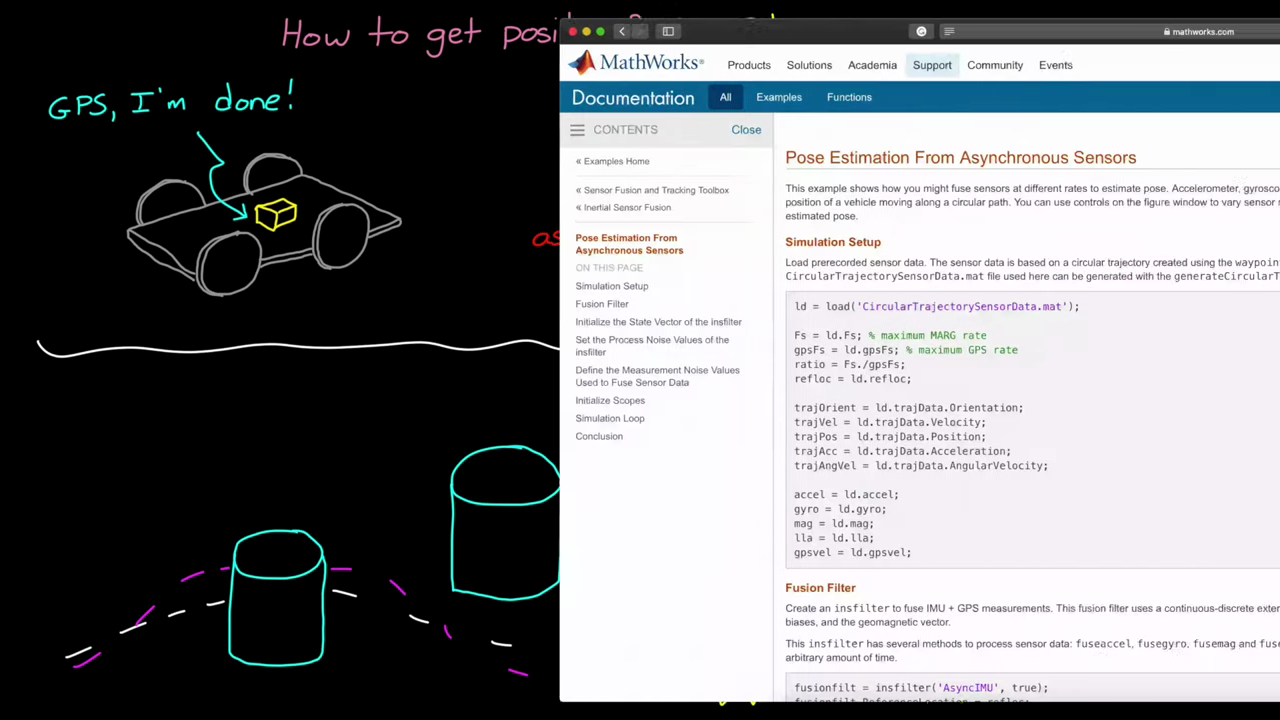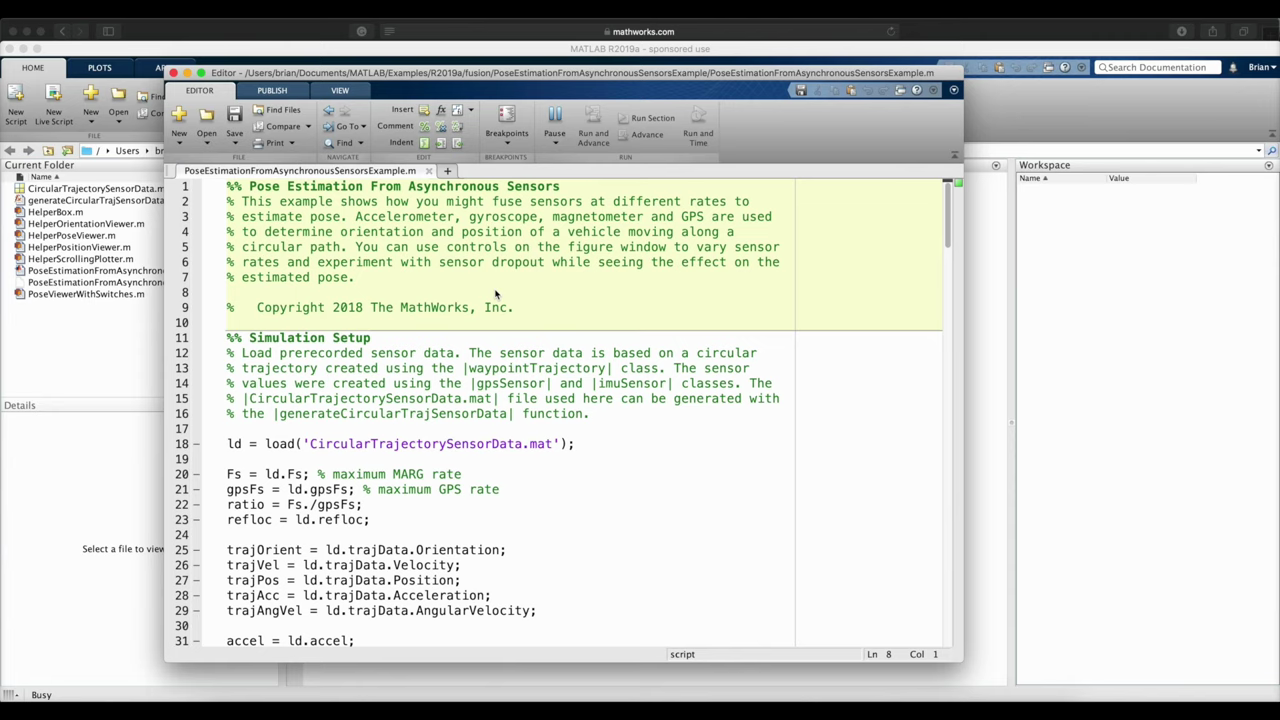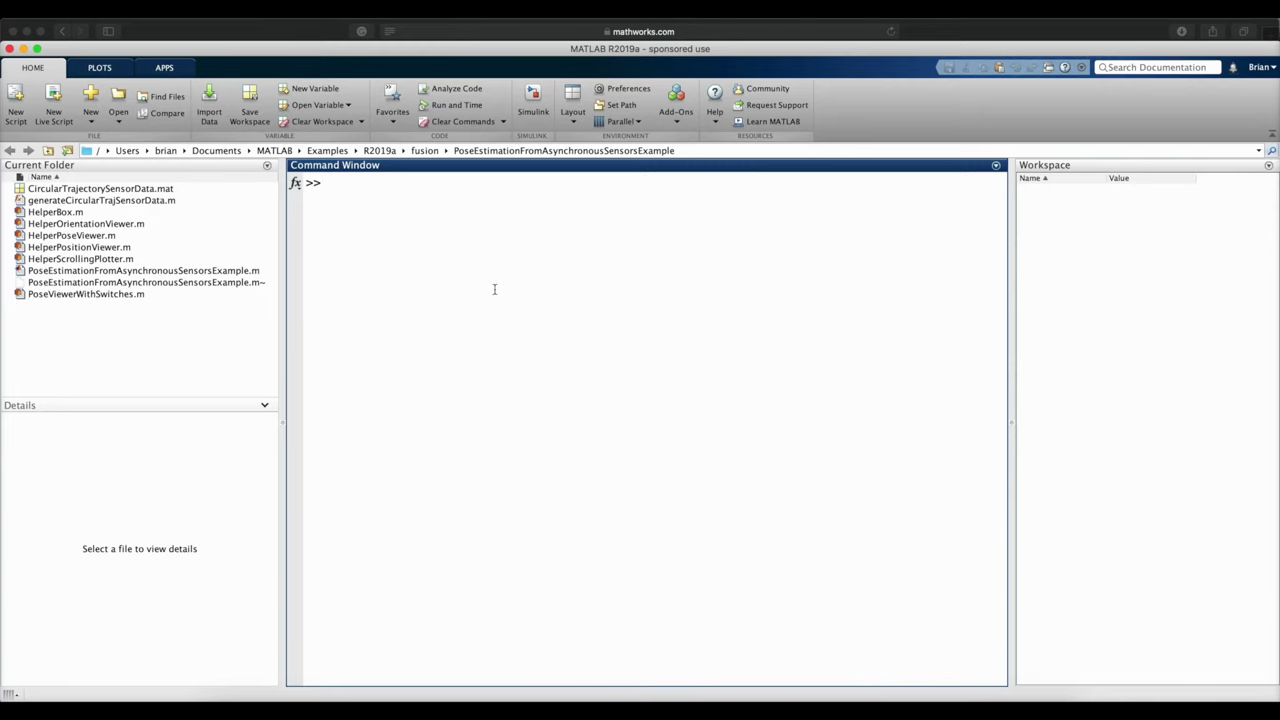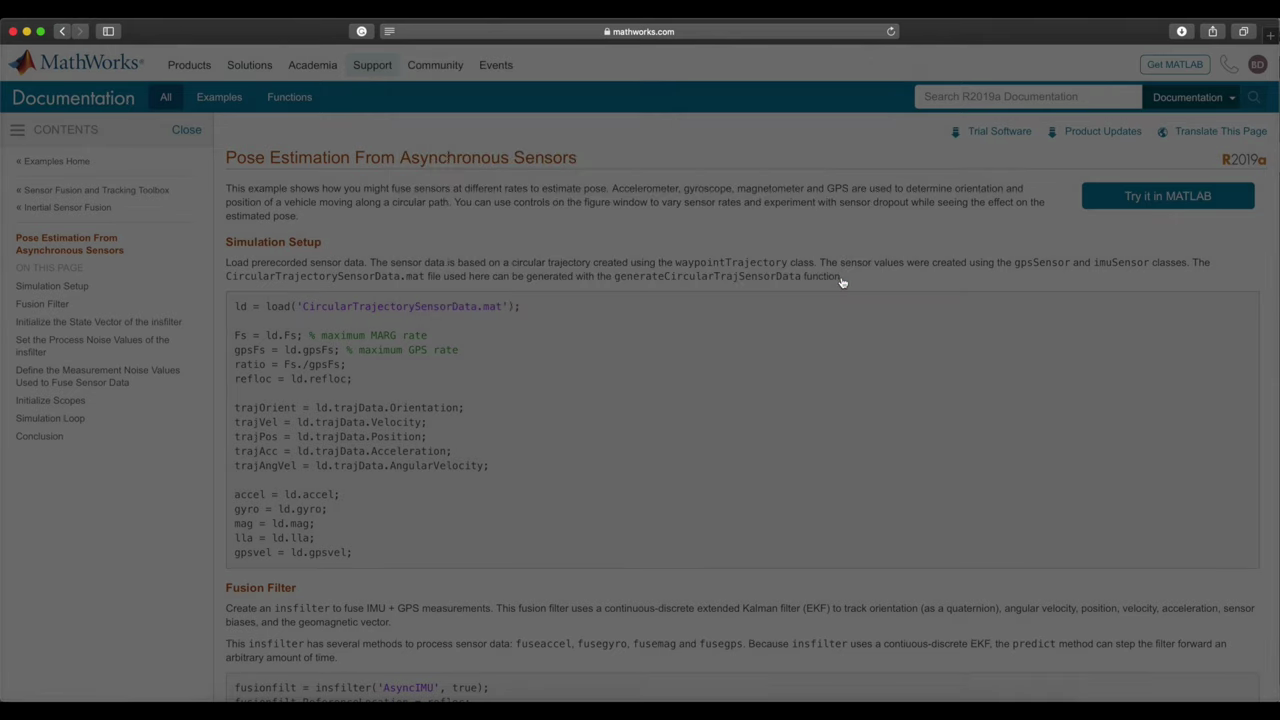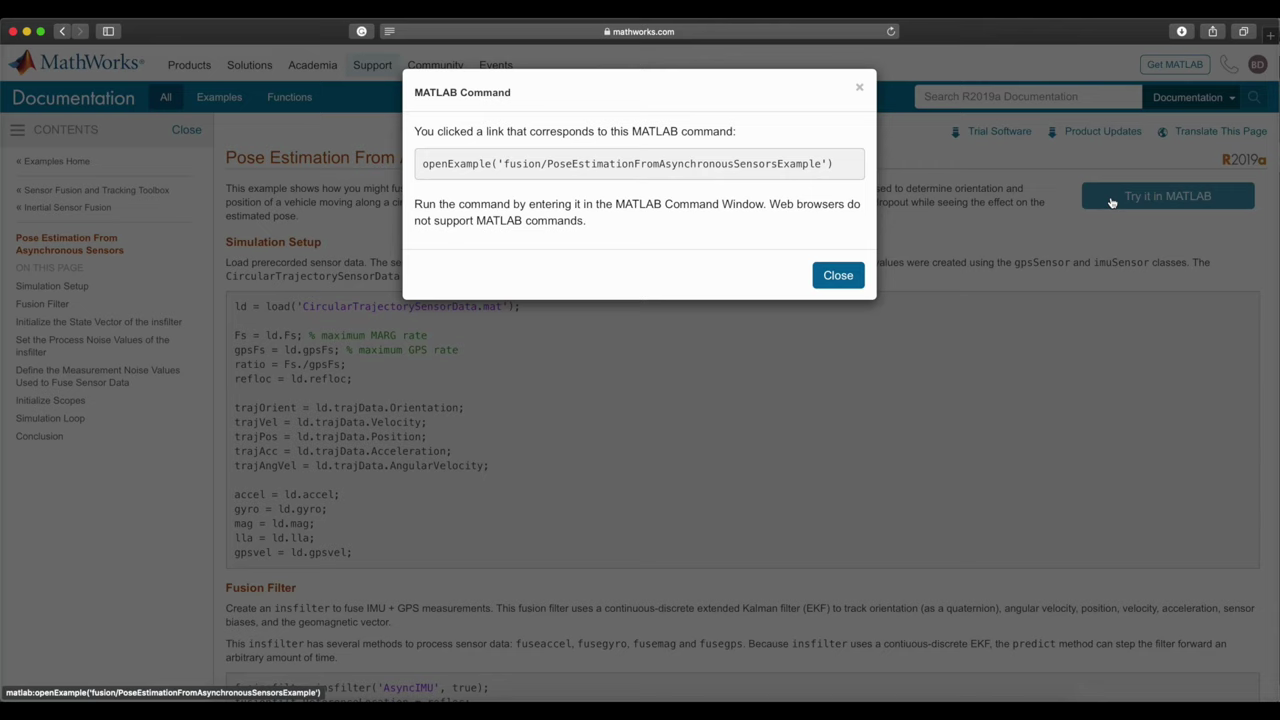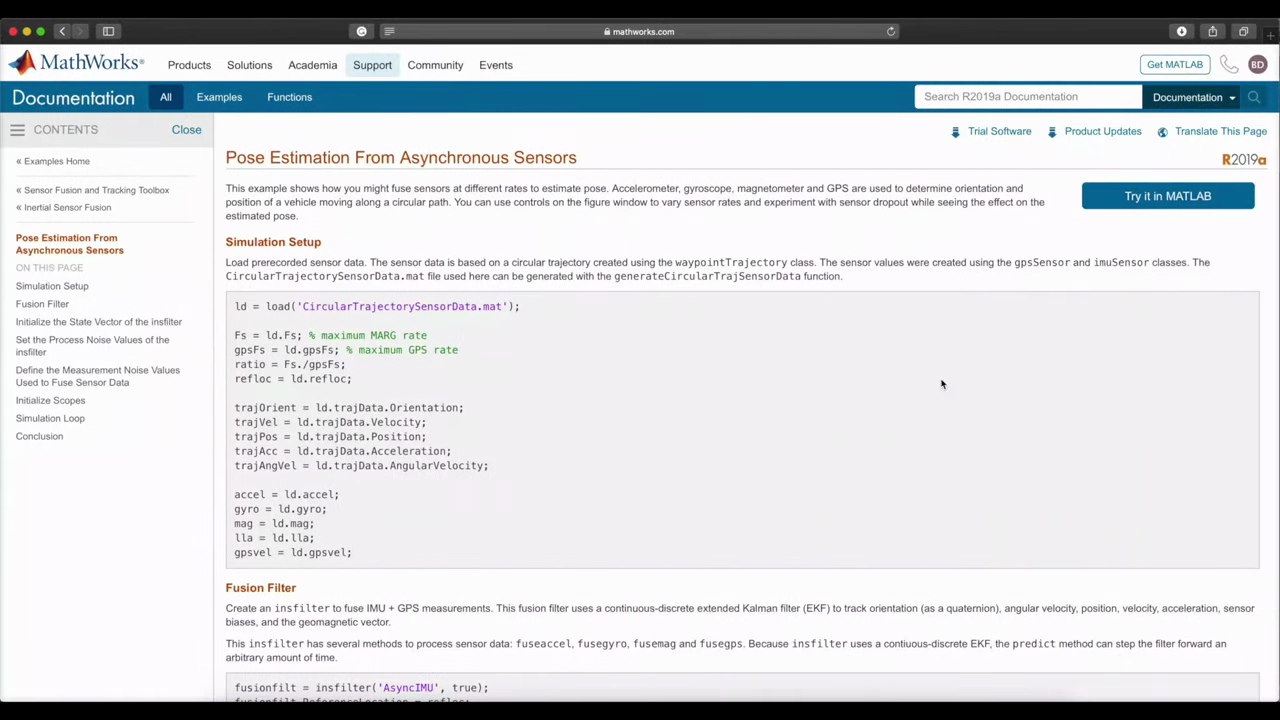This example uses a GPS, excell gyro and magnetometer to estimate which is both orientation and position, as well as a few other states. Now the script generates a true path and orientation profile that the system follows. The true orientation is the red cube and the true position is the red diamond. Show more

Show less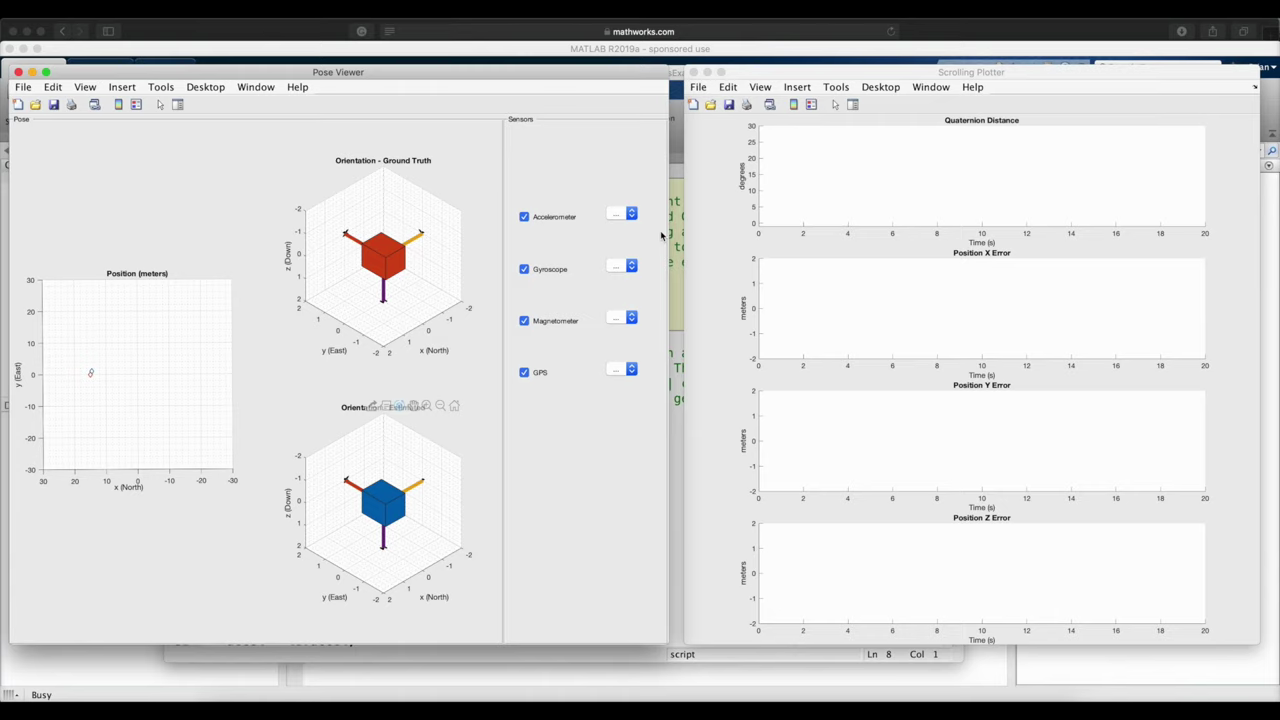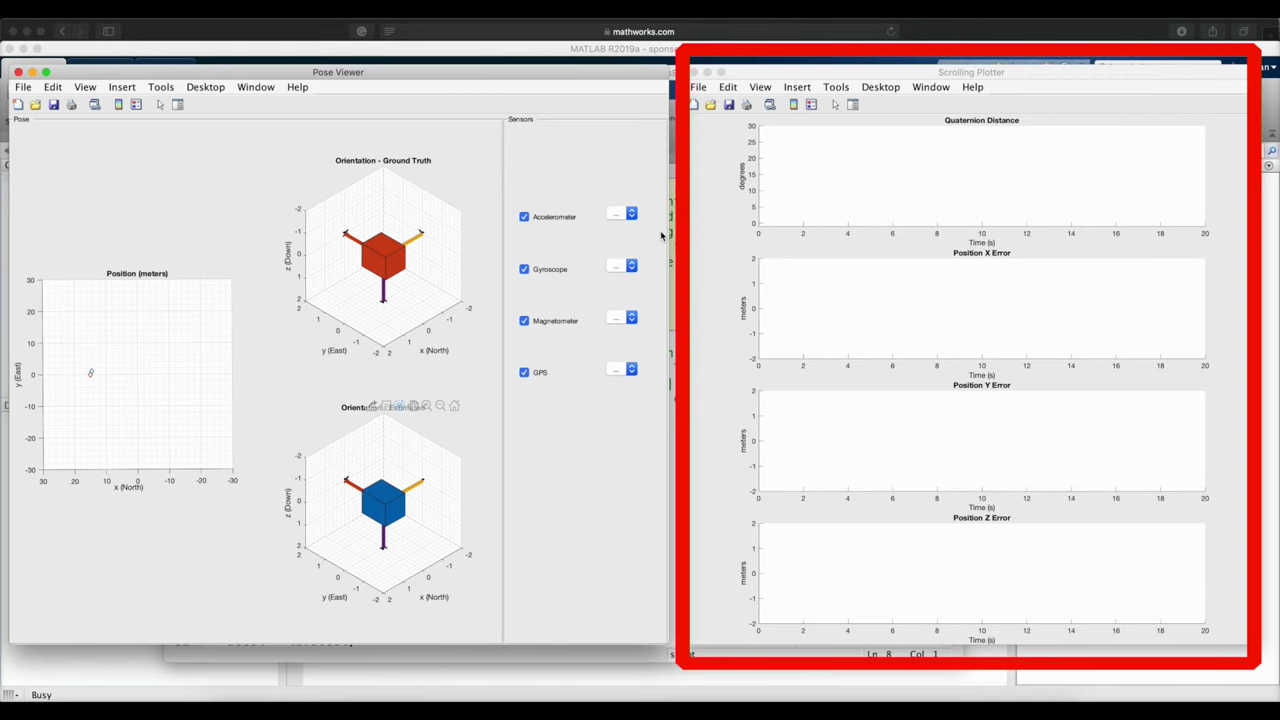Now the pose algorithm is using the available sensors to estimate orientation and position, and it shows the results of that as the blue cube and the blue diamond respectively. So that's what we want to watch. How closely do the blue objects follow the red objects? And the graph on the right plots the error, if you just want to see a more quantitative result. And the cool thing about this is that while the script is running, the interface allows us to change the sample rates of each of the sensors or remove them from the solution altogether, so that we can see how it impacts the estimation. Show more

Show less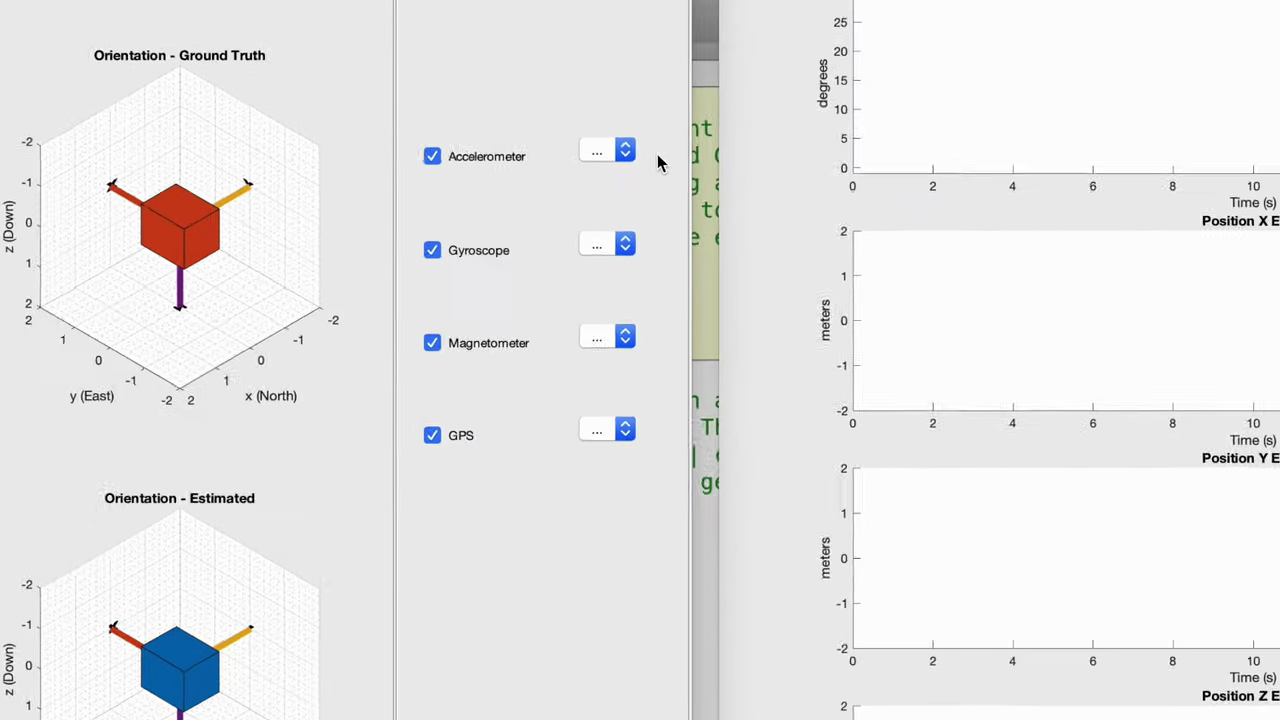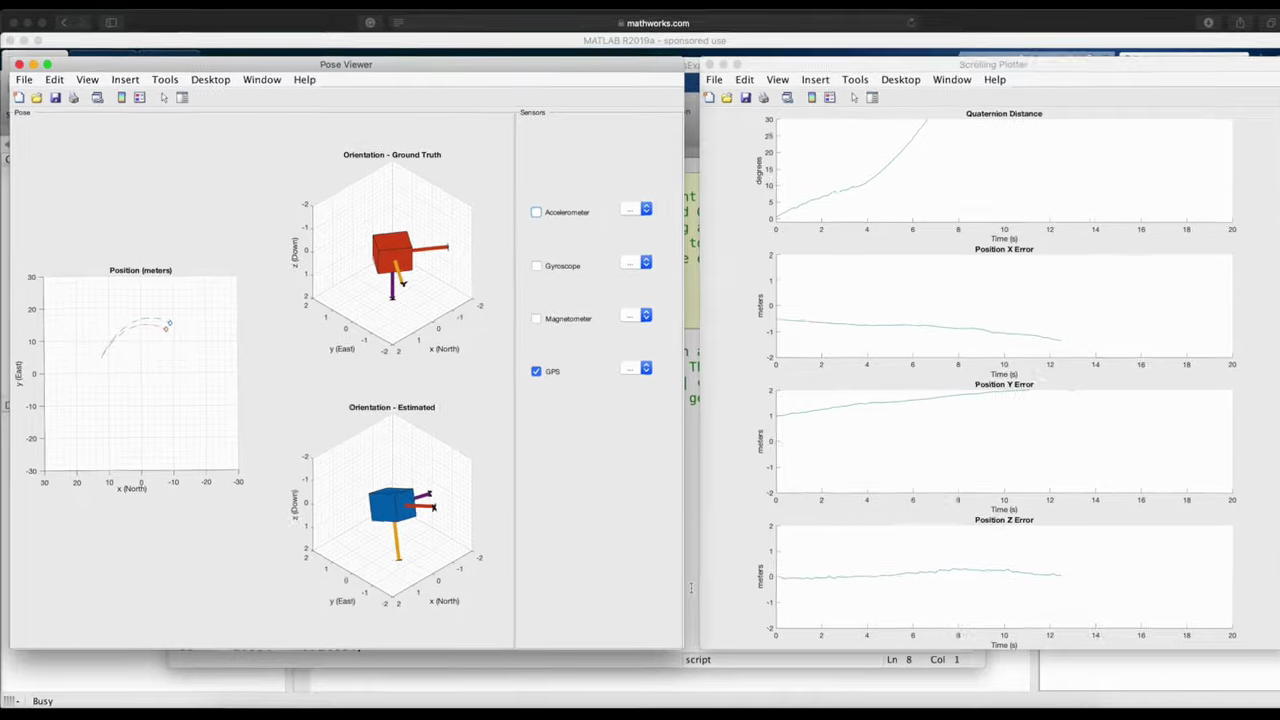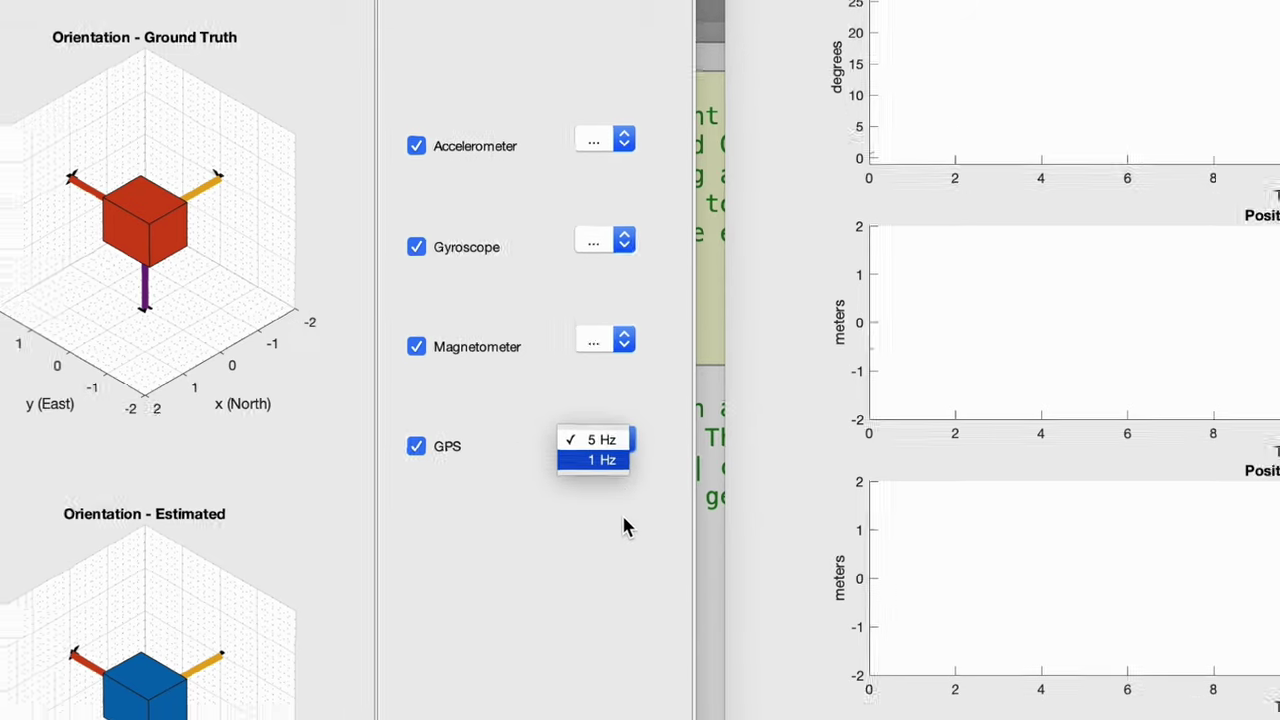So let's start by removing all of the sensors except for the GPS, and we'll read the GPS five times a second. The default trajectory in the script is to follow a circle with a radius of about 15 meters, and you can see that it's moving around this circle pretty slowly. Now the orientation estimate is way off, as you'd expect, since we don't have any orientation sensors active, but the position estimate isn't too bad. After the algorithm settles and removes that initial bias, we see position errors of around plus and minus 2 meters in each axis. So now let me add back in the IMU sensors and we can see if our result is improved. Show more

Show less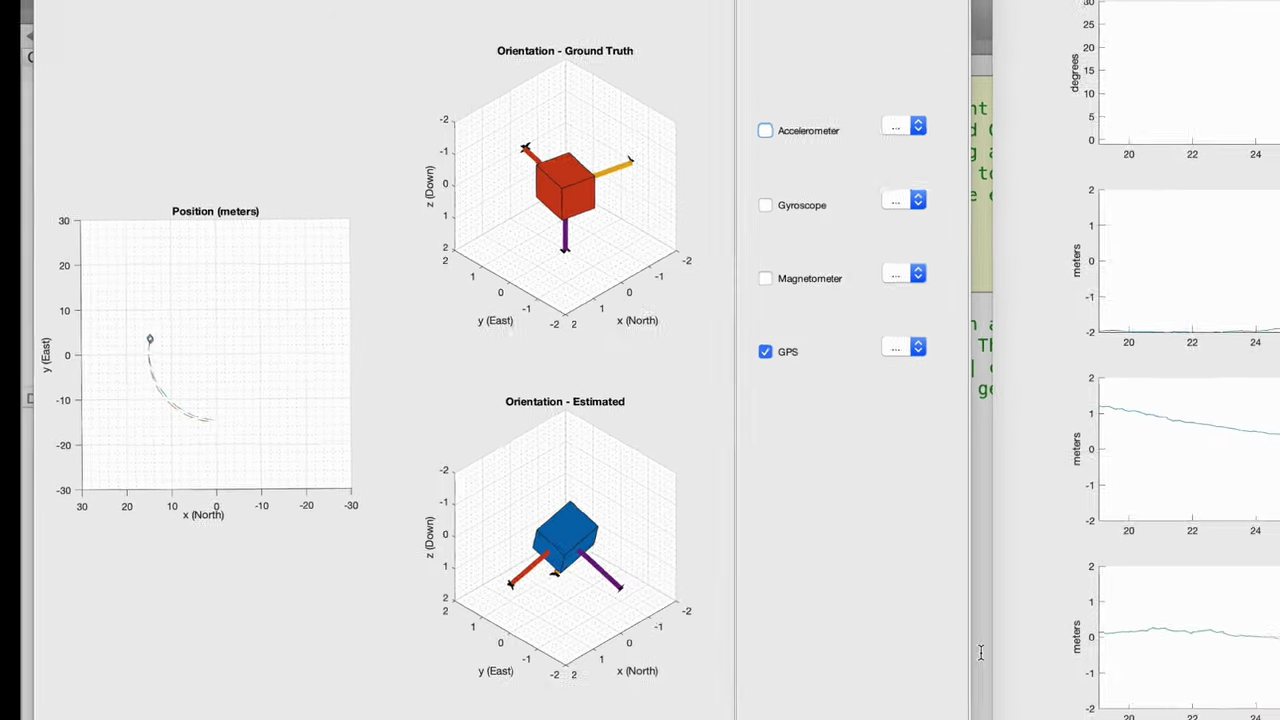Well, it's taking several seconds for the orientation to converge, but you can see that it's slowly correcting itself back to the true orientation. Also, the position estimate is: well, it's about the same, plus or minus two meters, maybe a little less than that. This is a relatively slow movement, and it's such a large trajectory that the IMU sensors that are modeled here are only contributing a minor improvement over the GPS alone. The GPS velocity measurement is enough to predict how the object moves over the point. Two seconds between measurements, since the object isn't accelerating too quickly. This setup is kind of analogous to using GPS to get directions from a map, your phone while you're driving. Adding those additional sensors from the IMU aren't really going to help too much. So now let's go in the opposite direction and create a trajectory that is much faster. In the trajectory generation script I'll just speed up the velocity of the object, going around the circle from two point five to twelve point five meters per second. Show more

Show less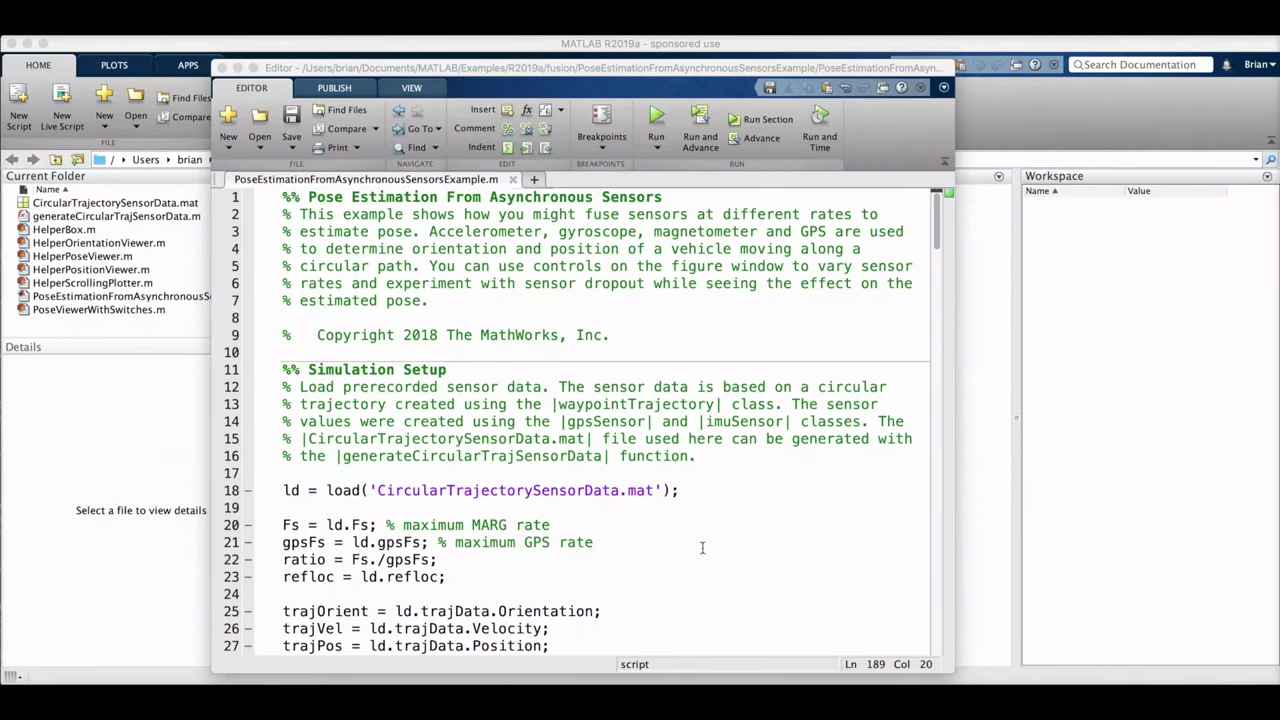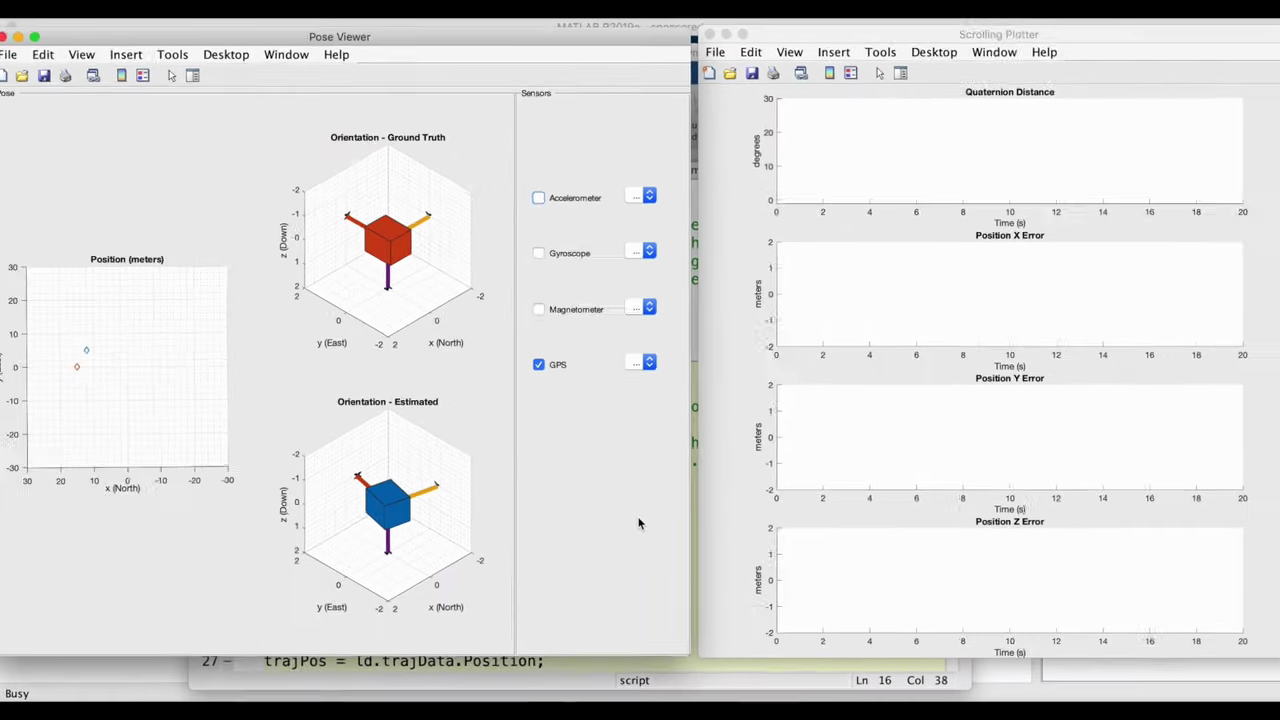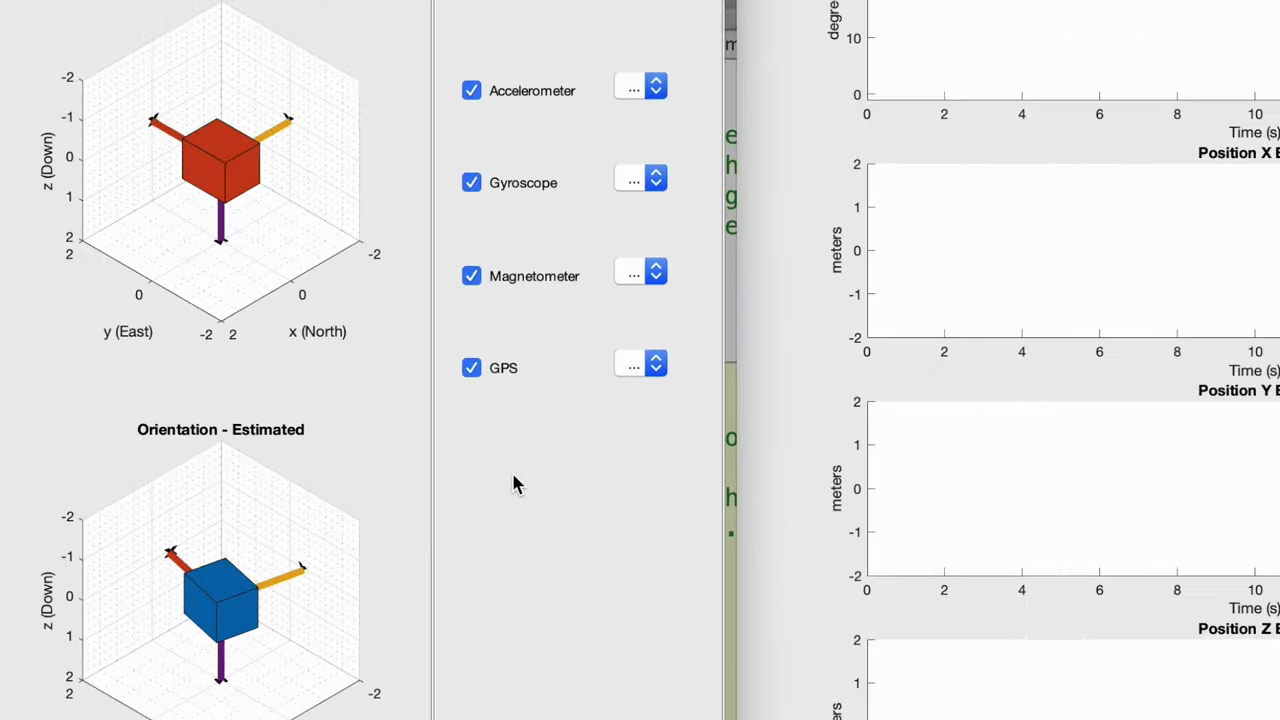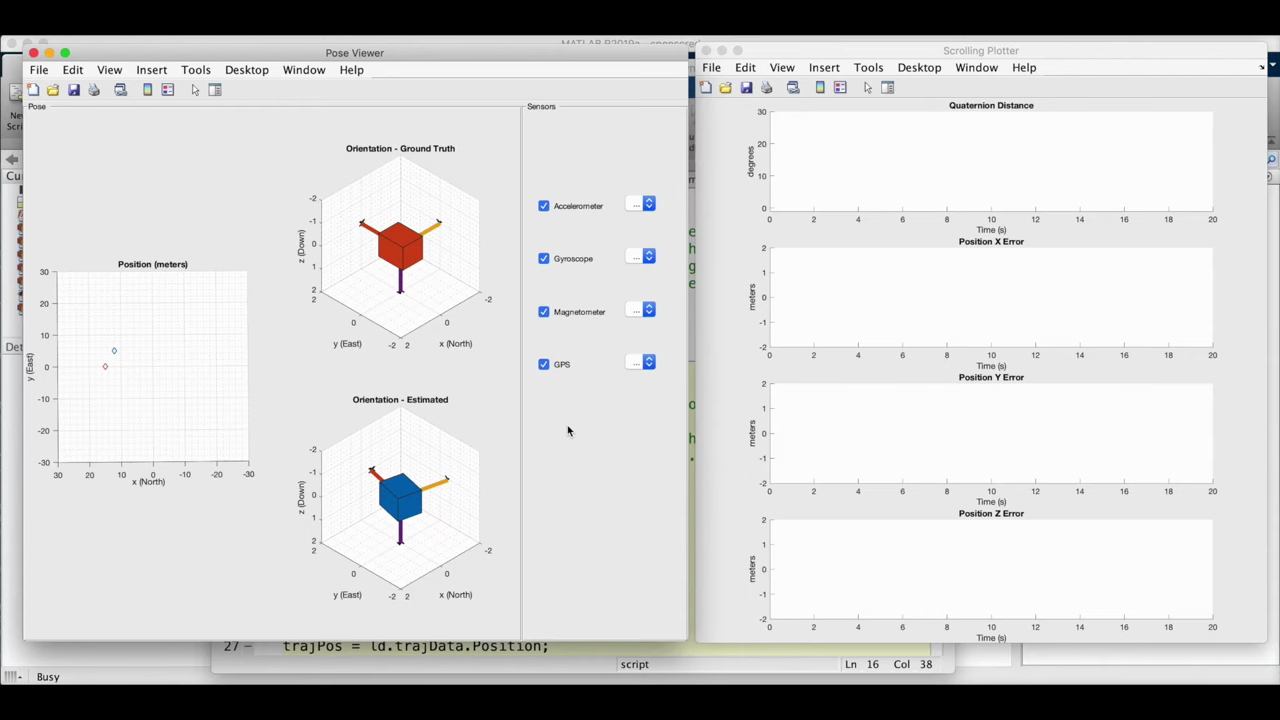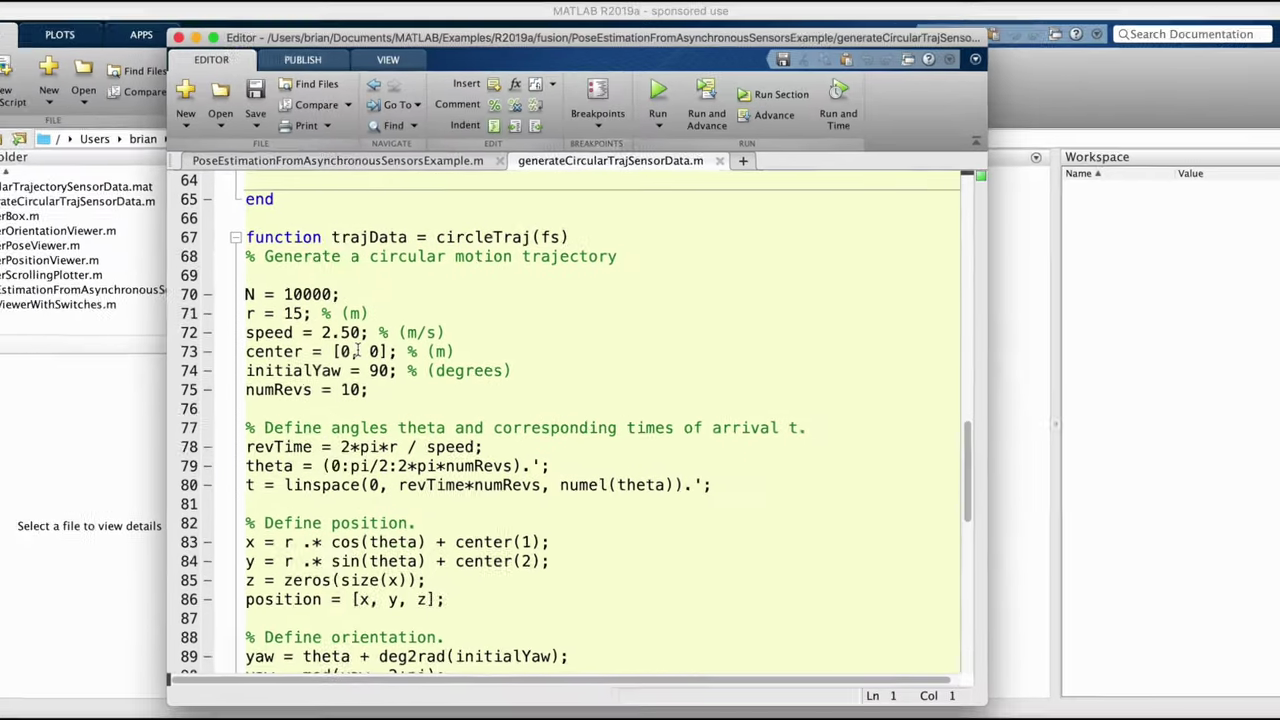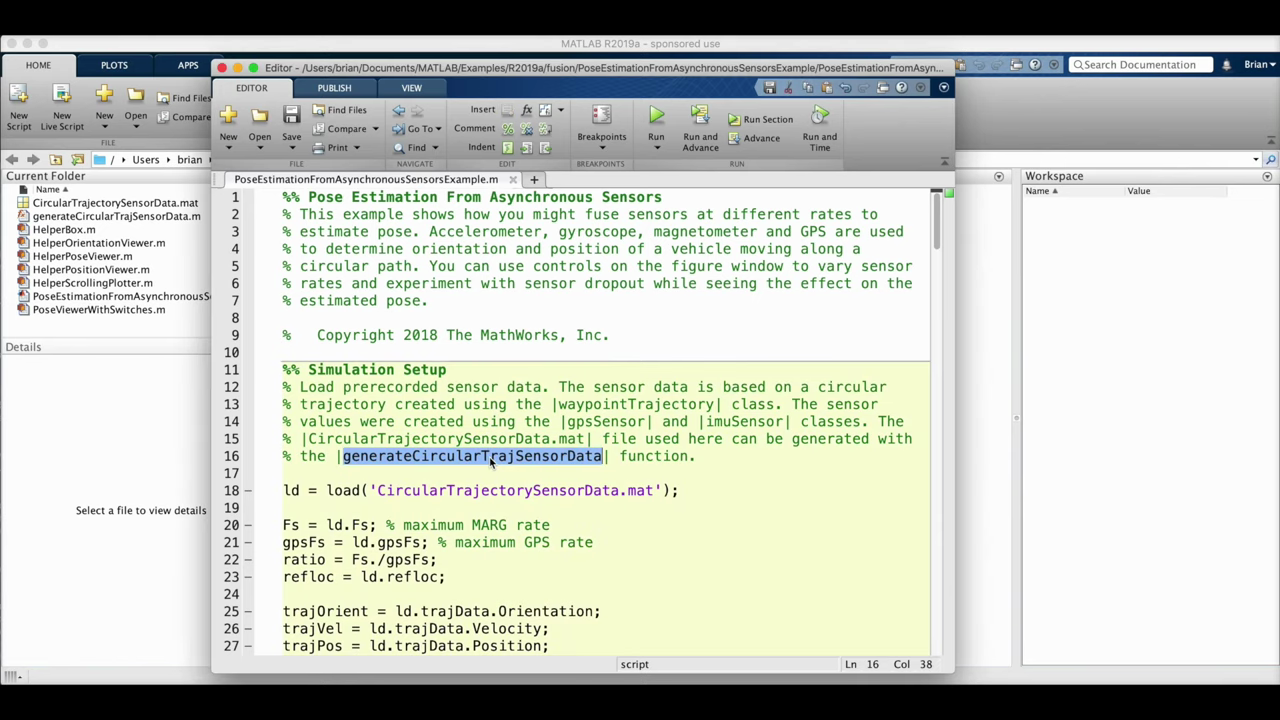This is going to create more angular acceleration in a shorter amount of time and, to really emphasize the point I'm trying to make here, I'm going to slow the GPS sample time down to once per second. So let's give this a shot, okay? So what's happening here is that when we get a GPS measurement, we get both position and velocity. So once a second we get a new position update that puts the estimate within a few meters of the truth, but we also get the current velocity, and so for one second the algorithm propagates that velocity forward to predict what the object is doing between measurements. Show more

Show less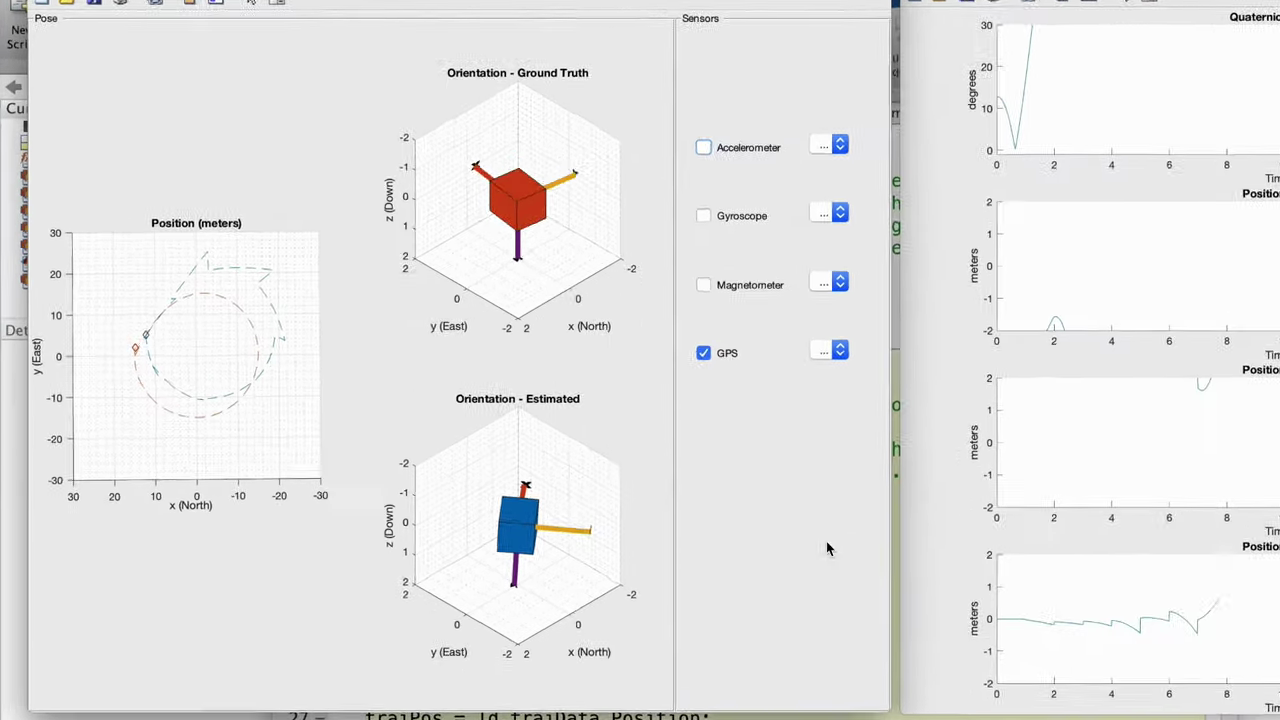And this works really well if the velocity is near constant for that one second, but poorly, as you can see, when the velocity is rapidly changing. This is the type of situation that is similar to a drone that has to make rapid turns and avoid obstacles, and it's here where the addition of the IMU will help, because we won't have to rely on propagating a static velocity. For one second. We can estimate velocity and rotation using the IMU sensors. Now to see the improvement, I've placed two different runs next to each other. The left is the GPS only that we just saw, and the right is with the addition of the IMU. You can see, at least visually, how the GPS with the IMU is different than the GPS alone. It's able to follow the position of the object more closely and creates a circular result rather than a saw blade. Show more

Show less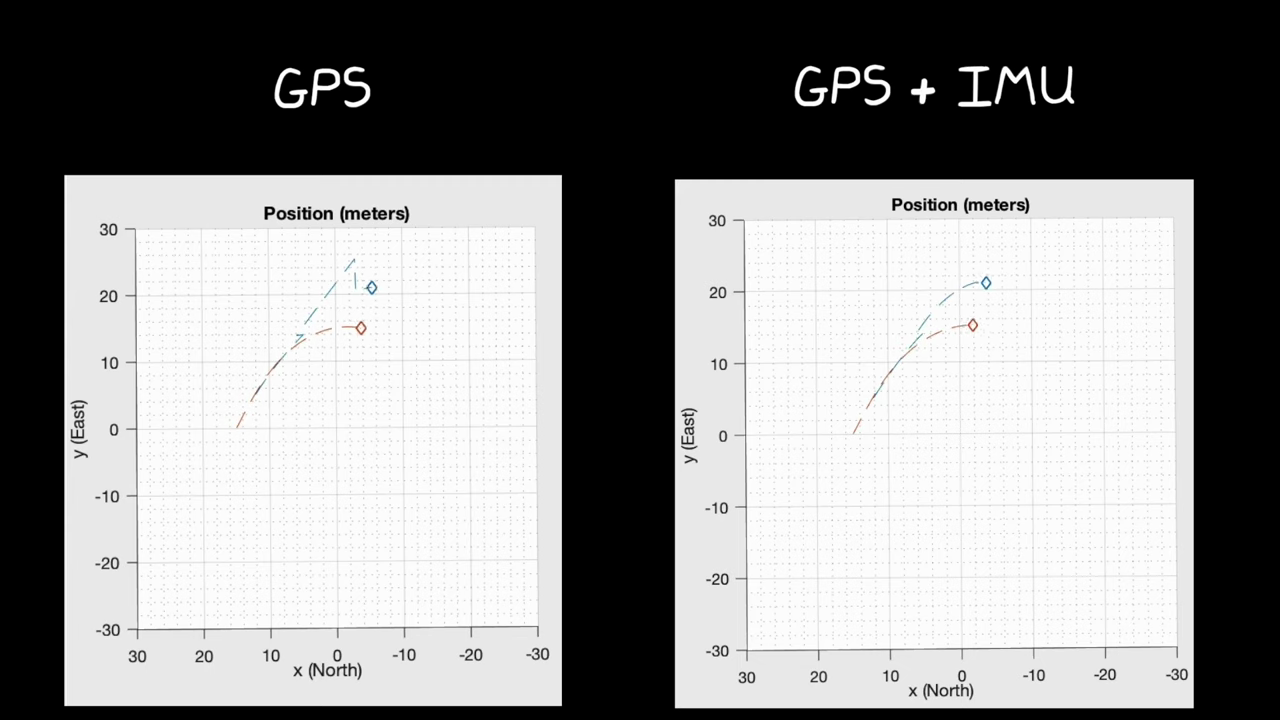Show less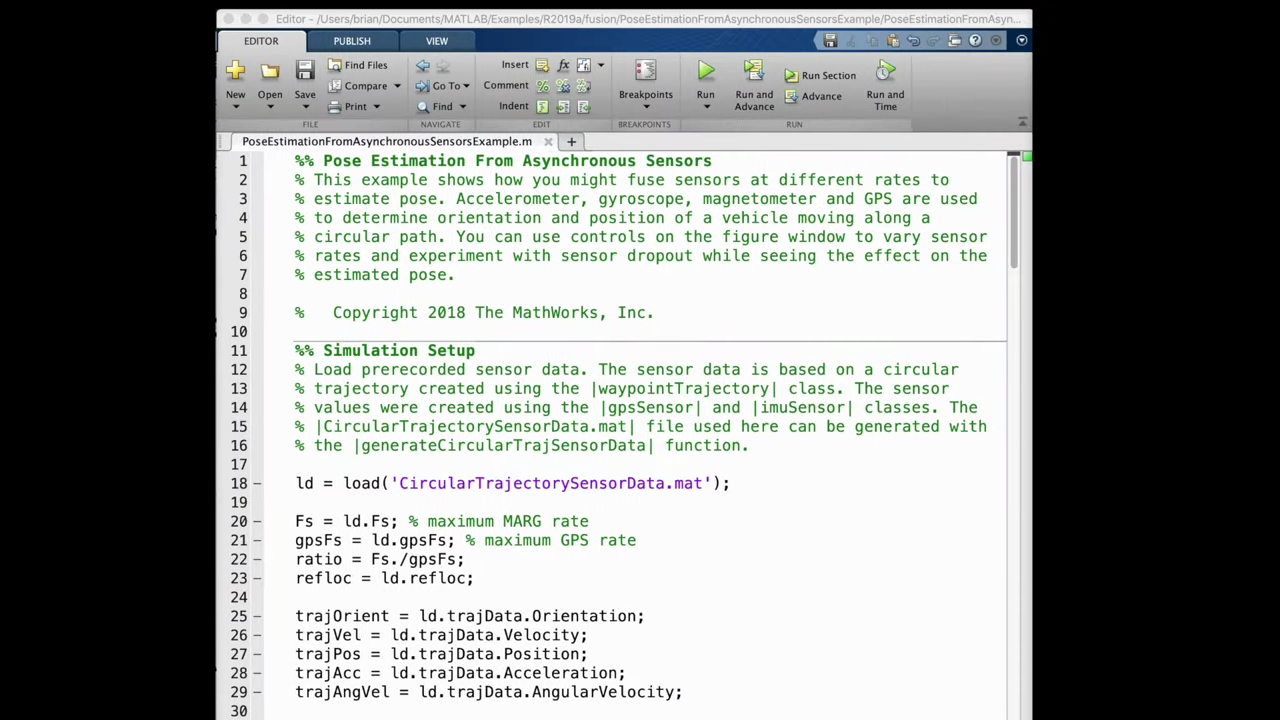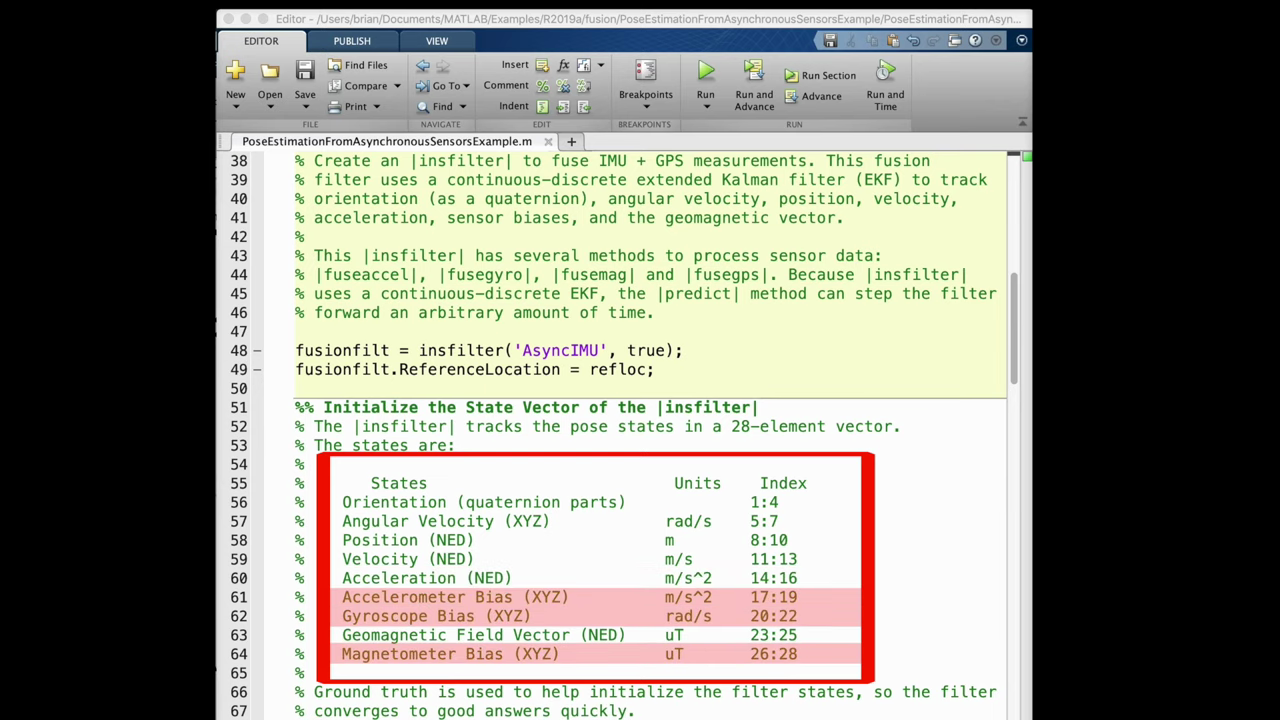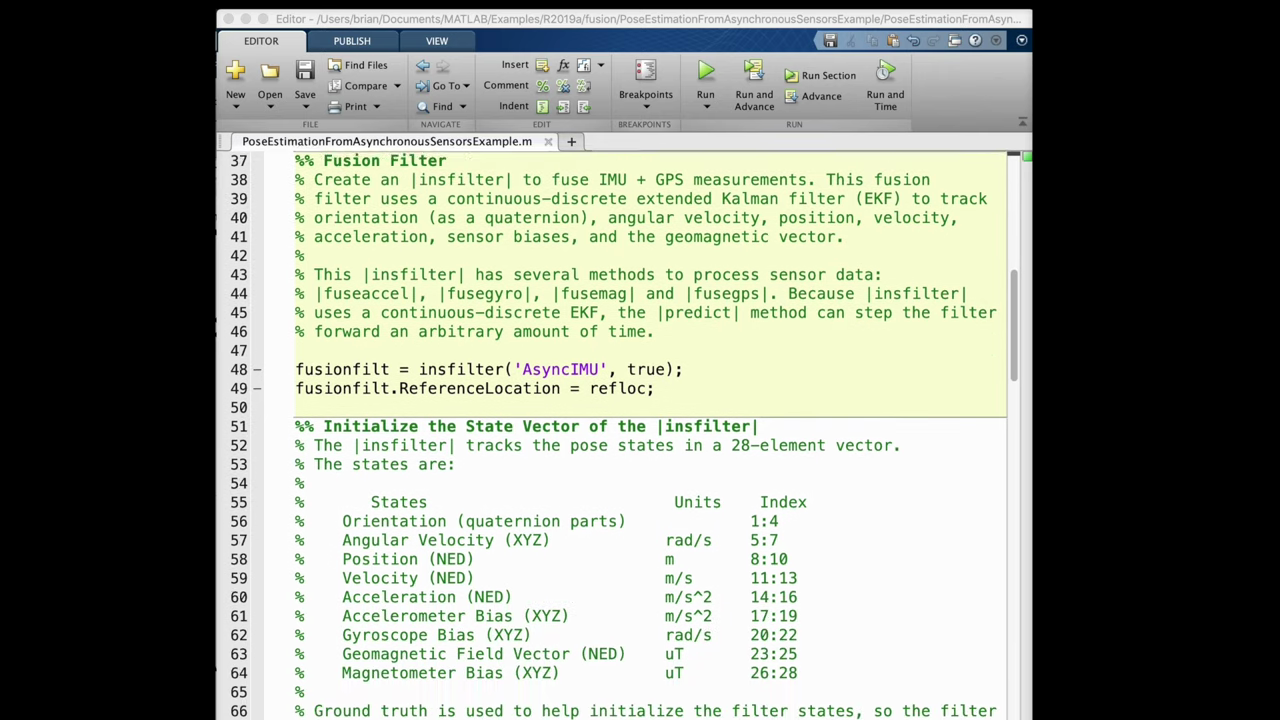You're gonna see below how this is handled, but the thing I want to point out here is that this is a massive common filter. The state vector has 28 elements in it that are being estimated simultaneously. There's the obvious states like orientation, angular velocity, linear position, velocity and acceleration. But the filter is also estimating the sensor biases and the mag field vector. Estimating sensor bias is extremely important because bias drifts over time. This means that even if you calculate sensor bias before you operate your system and you hard-code that calibration value into your software, it's not going to be accurate for long and any bias that we don't remove will be integrated and cause the estimate to walk away from the truth when we rely on that sensor. Show more

Show lessNow, if you don't have a good initial estimate of sensor bias when you start your system, then you can't just turn on your filter and Trust it right away. You have to give it some time to not just estimate the main states that you care about, like position and velocity, but also to estimate some of the secondary states, like bias. Usually you let the common filter converge on the correct solution when the system is stationary and not controlled, or maybe while you're controlling it using a different estimation algorithm, or maybe you just let it run and you don't really care that the system is performing poorly while the filter converges. But this is one of the things that you need to consider during initialization of your system. Now, another thing we need to talk about here is how to initialize the filter. This is an EKF and it can estimate state for nonlinear systems. It does this by linearizing the models around its current estimate and then using that linear model to predict the state into the future. So if the filter is not initialized close enough to the true state, the linearization process can be so far off that it causes the filter to never actually converge. Show more

Show lessNow this isn't really a problem for this example, because the ground truth is known in the simulation. So the filter is simply initialized to a state close to truth. But in a real system you need to think about how to initialize the filter when you don't know that truth. Now, often this can be done by just using the measurements from the sensors directly, like using the last GPS reading to initialize position and velocity and just using the gyro to initialize your angular rate and so on. All right, with the filter initialized we can start running it, and every common filter consists of the same two-step process: predict and correct. Show more

Show less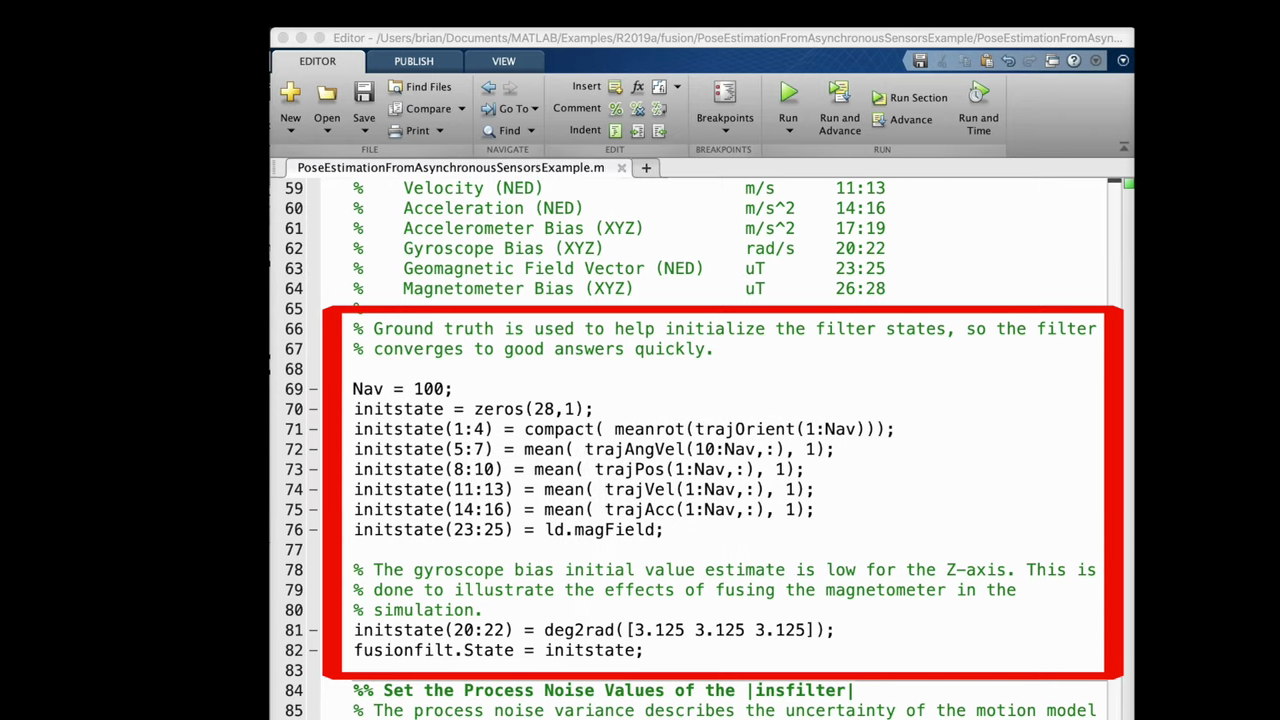To understand why we can think about it like this, if we want it to estimate the state of something, you know where it is or how fast it's going- there's two general ways to do this. We could just measure it directly, or we could use our knowledge of dynamics and kinematics and predict where it is. For example, imagine a car driving down the road and we want to know its location. We could use GPS to measure its position directly. That's one way. But if we knew where it started and its average speed, we could also predict where it'll be after a certain amount of time with some accuracy. And using those predictions alongside a measurement can produce a better estimate. So the question might be: why wouldn't we just trust our measurement completely here? It's probably better than our prediction. Well, as sort of an extreme example, what if you checked your watch and it said it was 3:00 pm? And then you waited a few seconds and checked it again and it said 4:00 pm. What you wouldn't automatically assume- an hour has passed just because your measurement said so. This is because you have a basic understanding of time, right, that is, you have this internal model that you can use to predict how much time has passed. And that would cause you to be sceptical of your watch if you thought seconds passed and it said an hour now, on the other hand, if you thought about an hour has passed, but the watch said 65 minutes. Show more

Show less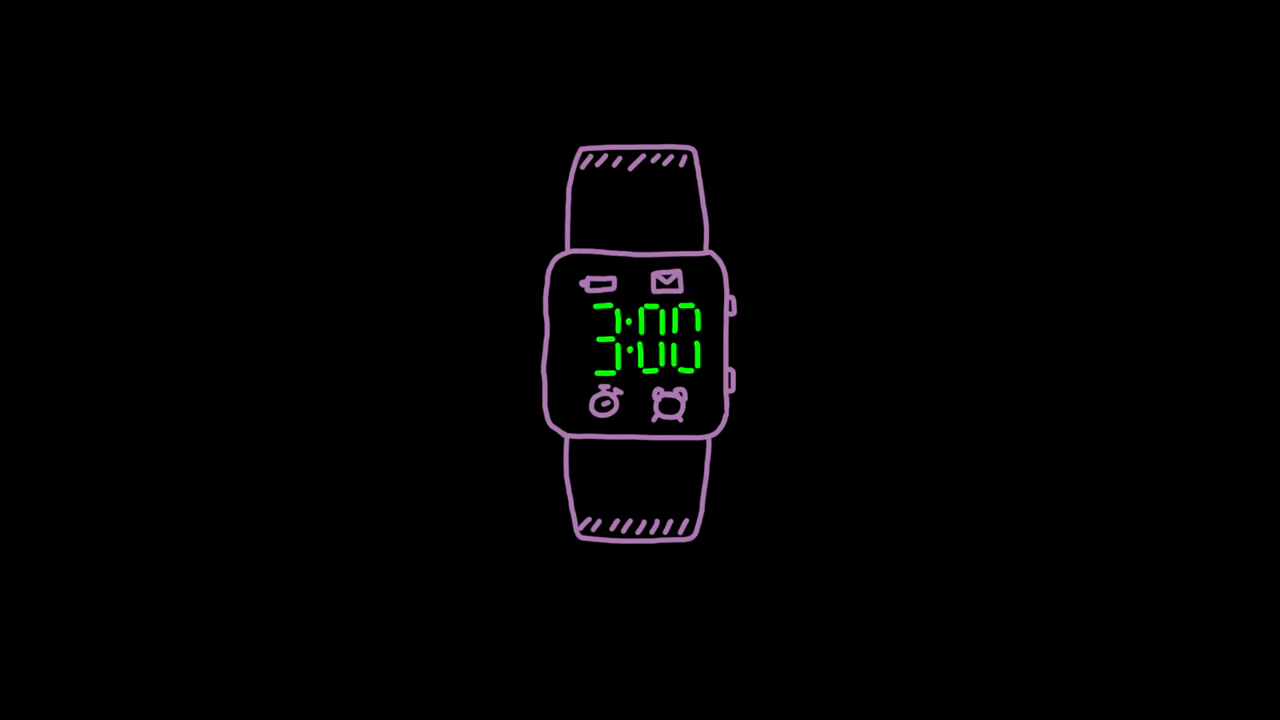You'd probably be more inclined to believe the watch over your own estimate, since you'd be less confident in your prediction. And this is precisely what a common filter is doing. It's predicting how the states will change over time based on a model that it has, and, along with the states, it's also keeping track of how trustworthy the prediction is based on the process noise that you've given it. And the longer the filter has to predict the state, the less confidence it has in the result. Then, whenever a new measurement comes in which has its own measurement noise associated with it, the filter compares the prediction with the measurement and then corrects its estimate based on the relative confidence in both. Show more

Show less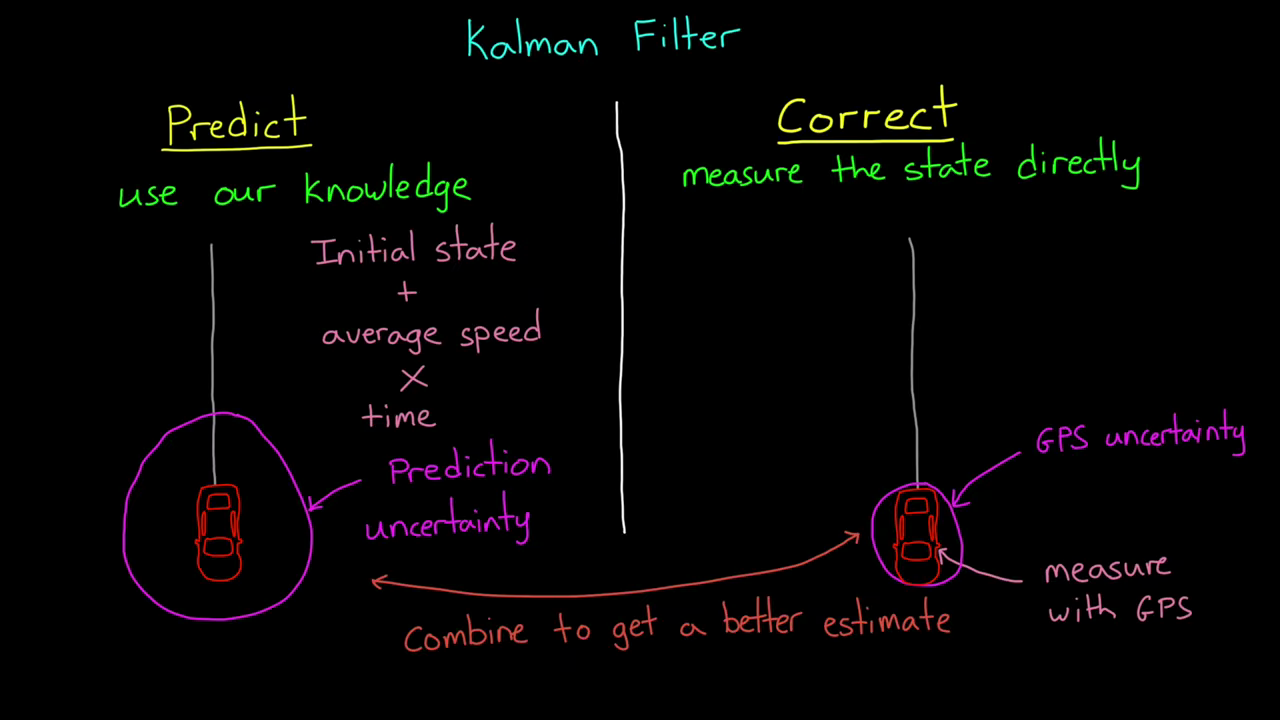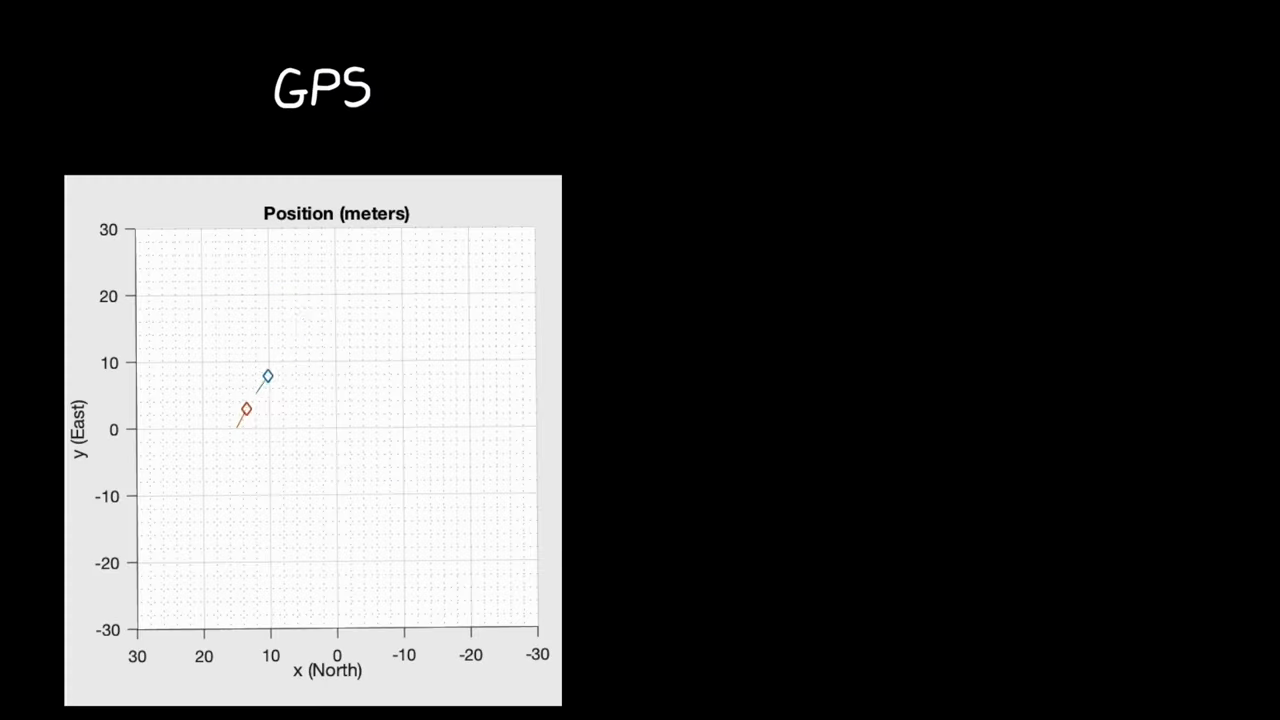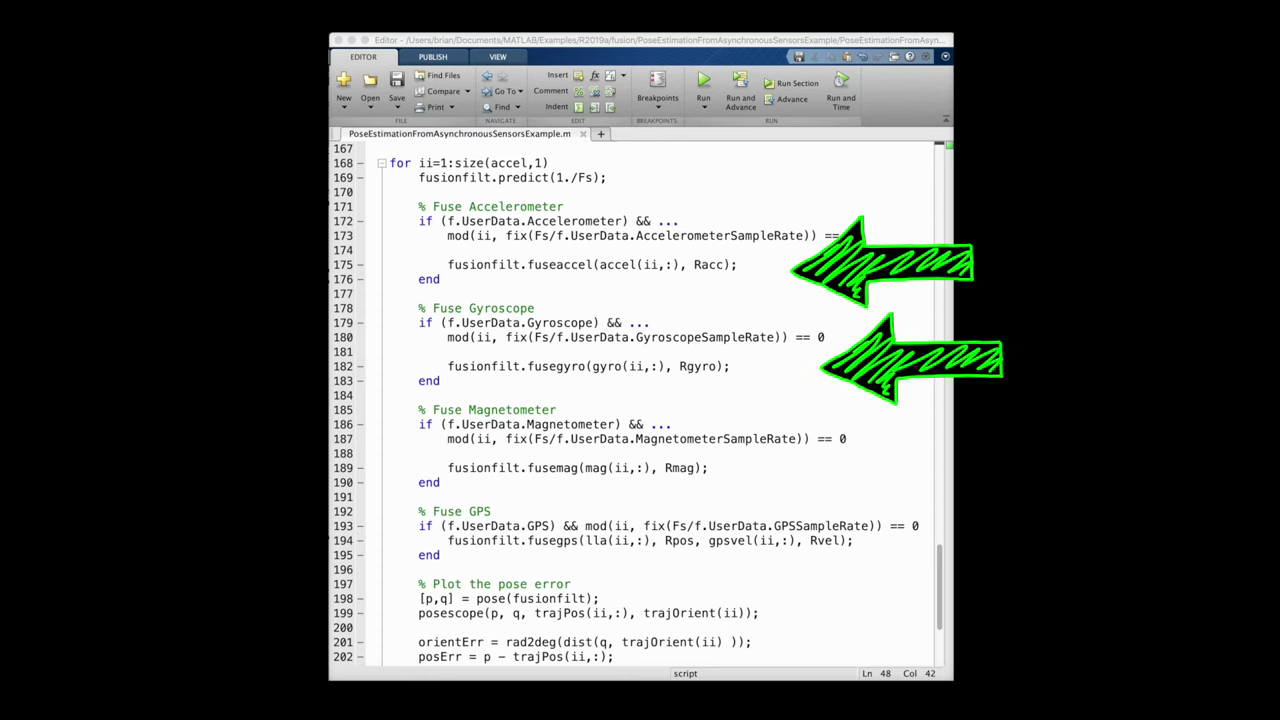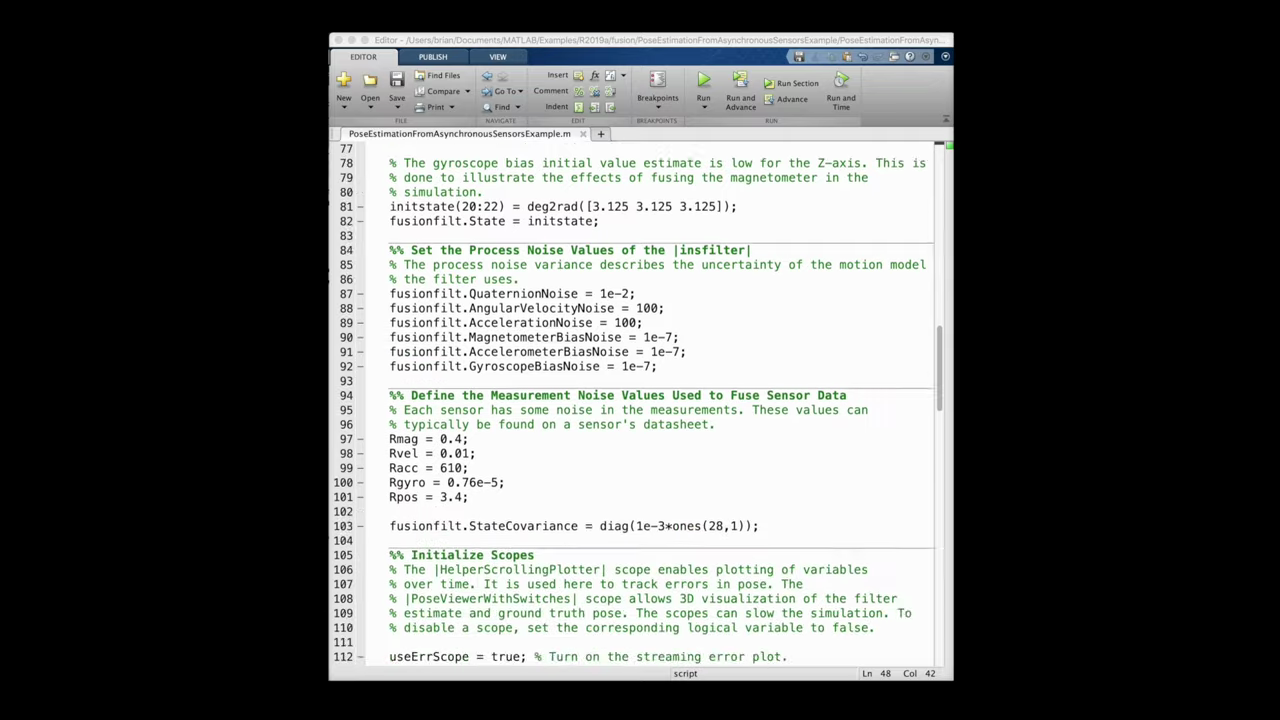And this is what the scripts doing. Also, the simulation runs at 100 Hertz and at every time step it predicts forward the estimate of the states and then, if there's a new measurement from any of the sensors, it runs the update portion of the common filter, adjusting the states based on the relative confidence in the prediction and the specific measurement. So it's in this way that the filter can run with asynchronous measurements. Now, with the GPS only solution that we started with, the prediction step could only assume that the velocity isn't changing over the one second and since there were no updates to correct that assumption, the estimate would drastically run away from truth. However, with the IMU, the filter is updating a hundred times a second and looking at the accelerometer in seeing that the velocity is in fact changing. So in this way the filter can react to a changing state faster with the quick updates of the IMU, then it can with the slower updates of the GPS and once the filter converges and it has a good estimate of sensor biases, then that will give us an overall better prediction and therefore a better overall state estimation. And this is the power of sensor fusion. Now I know this explanation might not have been perfectly clear and probably a bit fast, but I think it's hard to really grasp the topic by watching a video. Show more

Show less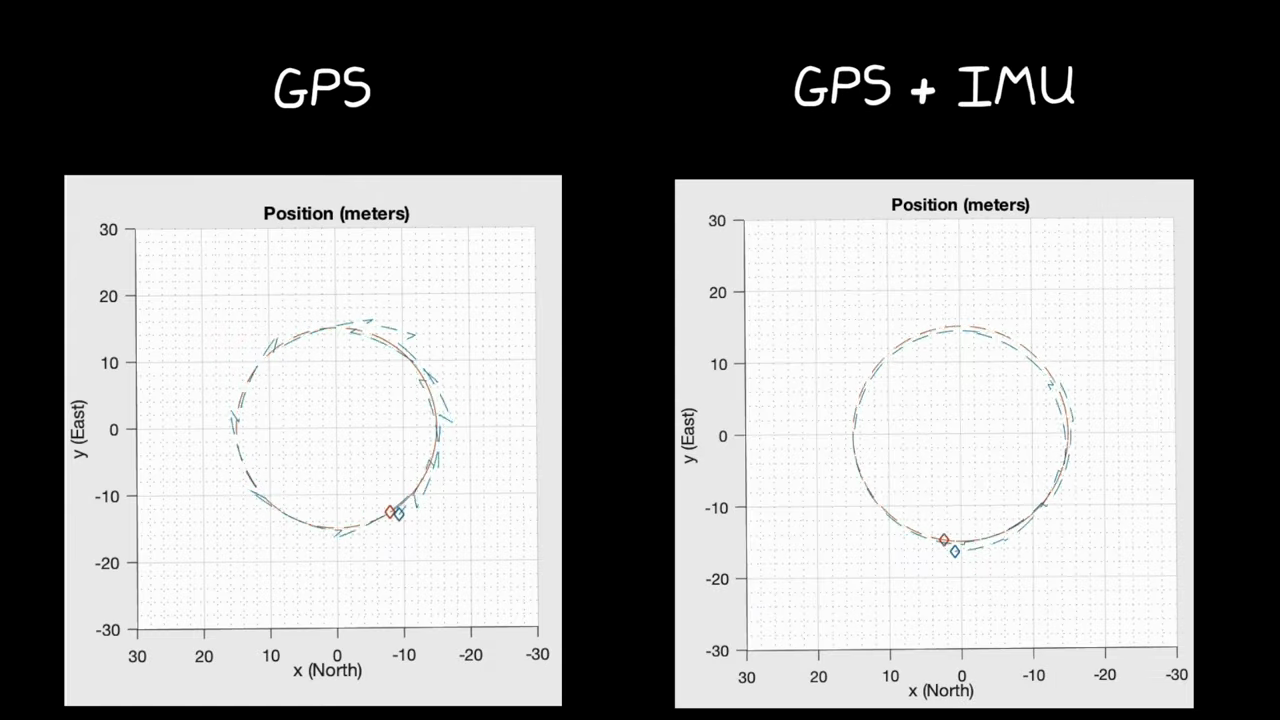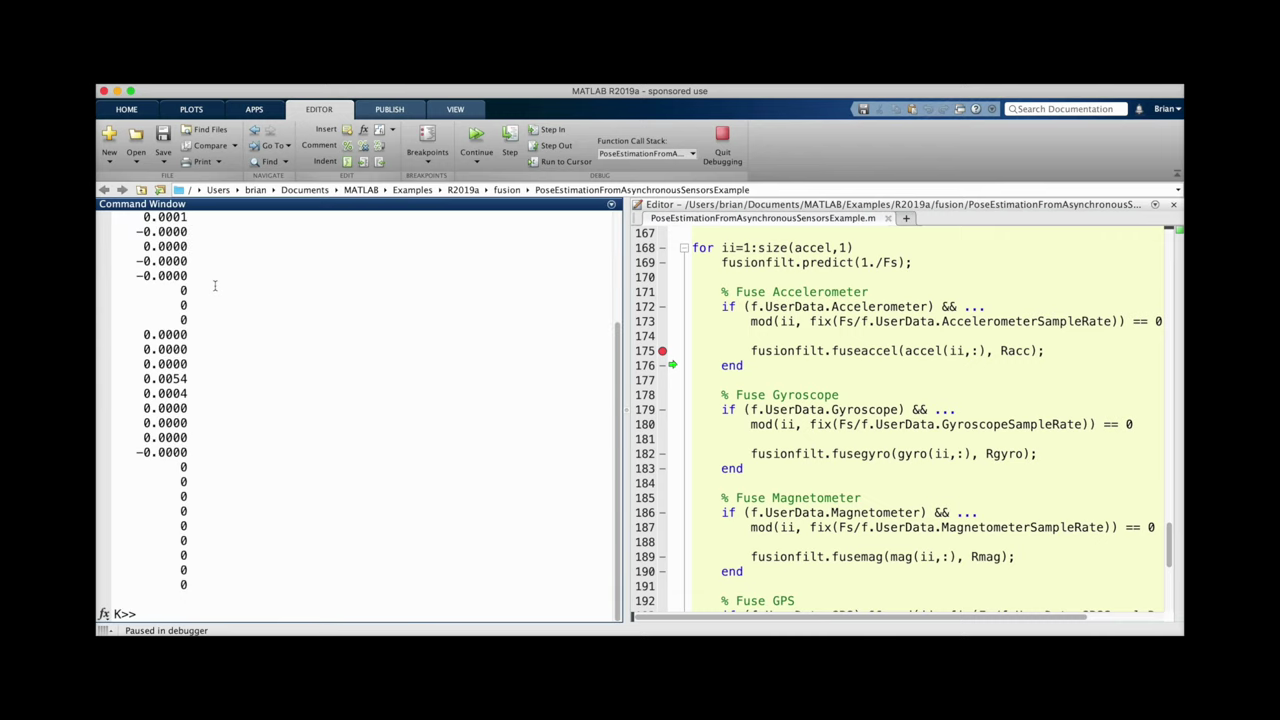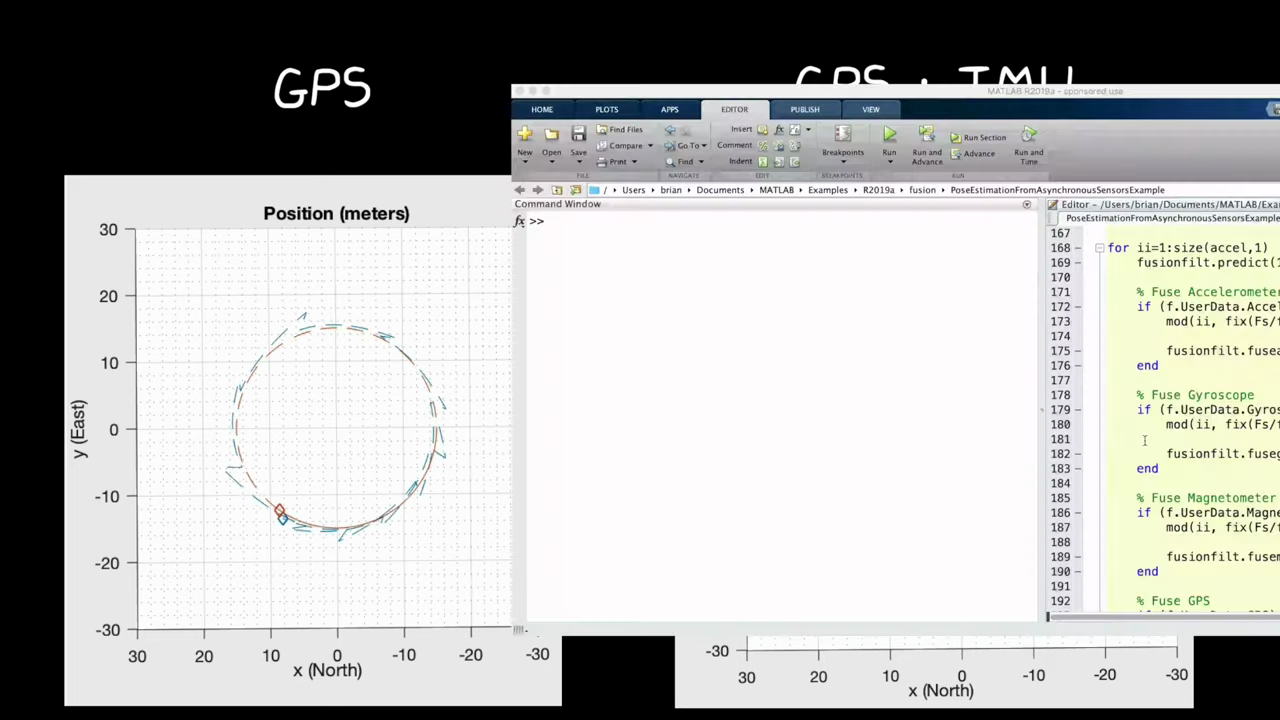So I would encourage you to play around with this example. You know, turn sensors on and off, change the rates, noise characteristics and the trajectory to see how the estimation is affected yourself. You can even dive further into the code and see how the EKF is implemented. I found it was helpful to place breakpoints and pause the execution of the script so that I could see how the different functions. Update the state vector. Okay, this is where I'm going to leave this. In the next video we'll start to look at estimating the state of other objects when we talk about tracking algorithms. So if you don't want to miss that in future Tech Talk videos, don't forget to subscribe to this channel and if you want, you can check out my channel control system lectures, where I cover more control theory topics there as well. Thanks for watching. Show more

Show less
Do you find this recap helpful? 👍 👎
Why?
Thank you for your feedback 😊